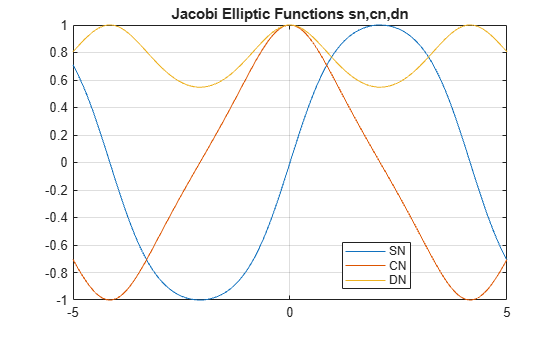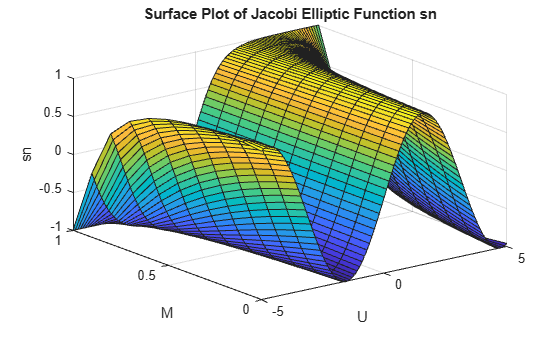# ellipj

Jacobi elliptic functions

## Syntax

``````[SN,CN,DN] = ellipj(U,M)``````
``````[SN,CN,DN] = ellipj(U,M,tol)``````

## Description

example

``````[SN,CN,DN] = ellipj(U,M)``` returns the Jacobi elliptic functions `SN`, `CN`, and `DN` evaluated for corresponding elements of argument `U` and parameter `M`. Inputs `U` and `M` must be the same size, or either `U` or `M` must be scalar.```

example

``````[SN,CN,DN] = ellipj(U,M,tol)``` computes the Jacobi elliptic functions to accuracy `tol`. The default value of `tol` is `eps`. Increase `tol` for a less accurate but more quickly computed answer.```

## Examples

collapse all

Find the Jacobi elliptic functions for `U = 0.5` and `M = 0.25`.

`[s,c,d] = ellipj(0.5,0.25)`
```s = 0.4751 ```
```c = 0.8799 ```
```d = 0.9714 ```

Plot the Jacobi elliptic functions for `-5≤U≤5` and `M = 0.7`.

```M = 0.7; U = -5:0.01:5; [S,C,D] = ellipj(U,M); plot(U,S,U,C,U,D); legend('SN','CN','DN','Location','best') grid on title('Jacobi Elliptic Functions sn,cn,dn')```Generate a surface plot of the Jacobi elliptic sn function for the allowed range of `M` and `-5≤U≤5`.

```[M,U] = meshgrid(0:0.1:1,-5:0.1:5); S = ellipj(U,M); surf(U,M,S) xlabel('U') ylabel('M') zlabel('sn') title('Surface Plot of Jacobi Elliptic Function sn')```The default value of `tol` is `eps`. Find the run time with the default value for arbitrary `M` using `tic` and `toc`. Increase `tol` by a factor of 1000 and find the run time. Compare the run times.

```tic ellipj(0.253,0.937)```
```ans = 0.2479 ```
`toc`
```Elapsed time is 0.687316 seconds. ```
```tic ellipj(0.253,0.937,eps*1000)```
```ans = 0.2479 ```
`toc`
```Elapsed time is 0.040418 seconds. ```

`ellipj` runs significantly faster when tolerance is significantly increased.

## Input Arguments

collapse all

Input array, specified as a scalar, vector, matrix, or multidimensional array. `U` is limited to real values. If `U` is nonscalar, `M` must be a scalar or a nonscalar of the same size as `U`.

Data Types: `single` | `double`

Input array, specified as a scalar, vector, matrix, or multidimensional array. `M` can take values 0≤ m ≤1. If `M` is a nonscalar, `U` must be a scalar or a nonscalar of the same size as `M`. Map other values of `M` into this range using the transformations described in , equations 16.10 and 16.11.

Data Types: `single` | `double`

Accuracy of result, specified as a nonnegative real number. The default value is `eps`.

Data Types: `single` | `double` | `int8` | `int16` | `int32` | `int64` | `uint8` | `uint16` | `uint32` | `uint64`

## Output Arguments

collapse all

Jacobi elliptic function sn, returned as a scalar, vector, matrix, or multidimensional array.

Jacobi elliptic function cn, returned as a scalar, vector, matrix, or multidimensional array.

Jacobi elliptic function dn, returned as a scalar, vector, matrix, or multidimensional array.

collapse all

### Jacobi Elliptic Functions

The Jacobi elliptic functions are defined in terms of the integral

`$u={\int }_{0}^{\varphi }\frac{d\theta }{\sqrt{1-m{\mathrm{sin}}^{2}\theta }}.$`

Then

Some definitions of the elliptic functions use the elliptical modulus k or modular angle α instead of the parameter m. They are related by

`${k}^{2}=m={\mathrm{sin}}^{2}a.$`

The Jacobi elliptic functions obey many mathematical identities. For a good sample, see .

## Algorithms

`ellipj` computes the Jacobi elliptic functions using the method of the arithmetic-geometric mean of . It starts with the triplet of numbers

`ellipj` computes successive iterations using

`$\begin{array}{l}{a}_{i}=\frac{1}{2}\left({a}_{i-1}+{b}_{i-1}\right)\\ {b}_{i}={\left(}^{{a}_{i-1}}\\ {c}_{i}=\frac{1}{2}\left({a}_{i-1}-{b}_{i-1}\right).\end{array}$`

Next, it calculates the amplitudes in radians using

`$\mathrm{sin}\left(2{\varphi }_{n-1}-{\varphi }_{n}\right)=\frac{{c}_{n}}{{a}_{n}}\mathrm{sin}\left({\varphi }_{n}\right),$`

being careful to unwrap the phases correctly. The Jacobian elliptic functions are then simply

`$\begin{array}{l}sn\left(u\right)=\mathrm{sin}{\varphi }_{0}\\ cn\left(u\right)=\mathrm{cos}{\varphi }_{0}\\ dn\left(u\right)=\sqrt{1-m\cdot sn{\left(u\right)}^{2}}.\end{array}$`

 Abramowitz, M. and I. A. Stegun, Handbook of Mathematical Functions, Dover Publications, 1965, 17.6.